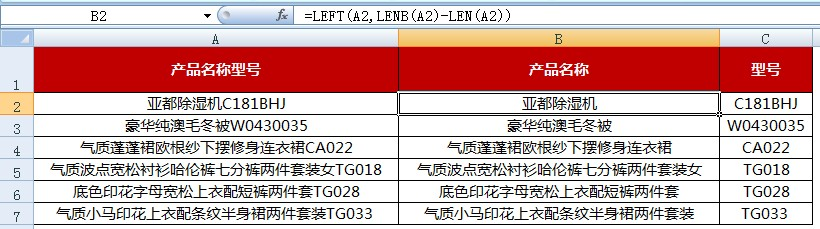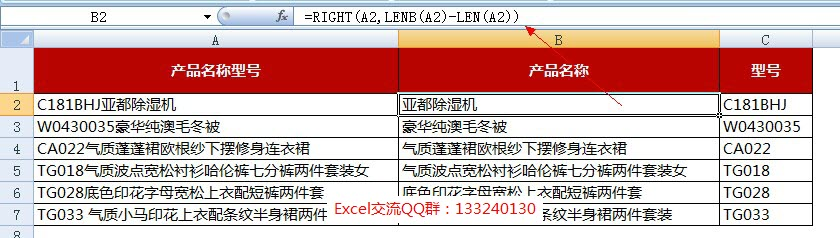# len函数和lenb函数实战案例：将excel 汉字和字母分开

[日期：2015-09-17]   来源：IT部落窝  作者：IT部落窝   阅读：498[字体： ]

本文为大家分享一个案例：excel 汉字和字母分开。如下图所示：将A列的产品名称和型号分开放在B列和C列。其实就是len函数和lenb函数的实操应用。excel 汉字和字母分开，本文我们分两种情况进行分享。

我们在B2单元格输入公式：=LEFT(A2,LENB(A2)-LEN(A2)) ，然后在C2单元格输入公式：=SUBSTITUTE(A2,B2,)，最后下拉复制公式，就实现了excel中汉字和字母分开。我们在B2单元格输入公式：=RIGHT(A2,LENB(A2)-LEN(A2))，然后在C2单元格输入公式：=SUBSTITUTE(A2,B2,)，同样下拉复制公式，也实现了excel中汉字和字母分开。
总结：上面的案例，其实就是LEN函数和LENB函数的应用。关于这两个函数，如果同学不清楚，可以参考之前的教程：http://www.ittribalwo.com/article/1146.html学习。

最后，为了让同学们更加清楚函数len和lenb的区别，我们分享出4个小案例。

len函数和lenb函数案例一：计算字数
计算A1单元格的字数，公式为：=Len(A1)
统计A2:A10区域内总字符数（数组公式）：{=Sum(Len(A2:A10))}

len函数和lenb函数案例二：截取字符串
从左截取除最右3位之外的字符串，ABCDE截取后为AB，公式为：=Left(A1,Len(A1)-3)

len函数和lenb函数案例三：统计个数
判断一个字符串中某个字符的个数，公式为：=Len(A1) - Len(Substitute(A1,"-",""))

len函数和lenb函数案例四：控制输入单元格字符输入
某列只能输入18位或15位长度字符：数据 - 数据有效性 - 允许：自定义。在下面框中输入公式：=Or(Len(C1)=15,Len(C1)=18)分享到： QQ空间 新浪微博 腾讯微博 人人网